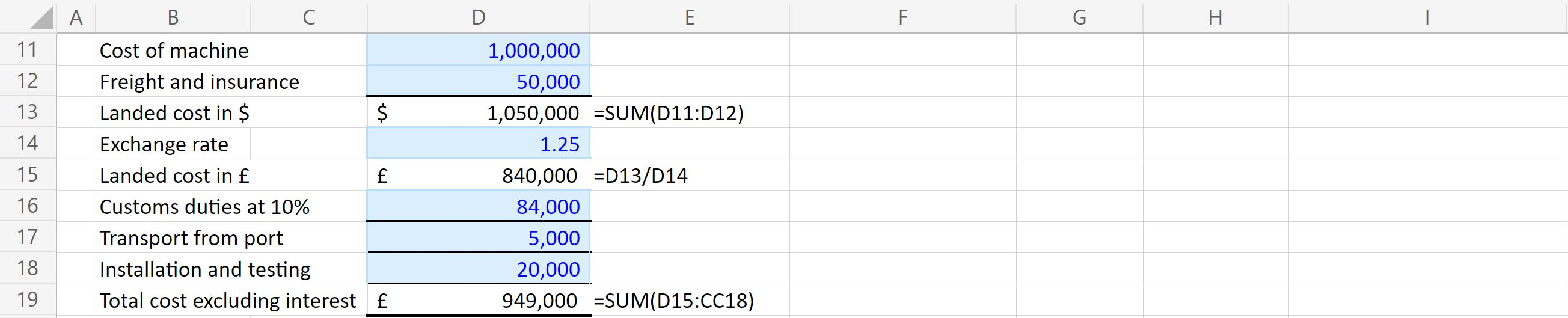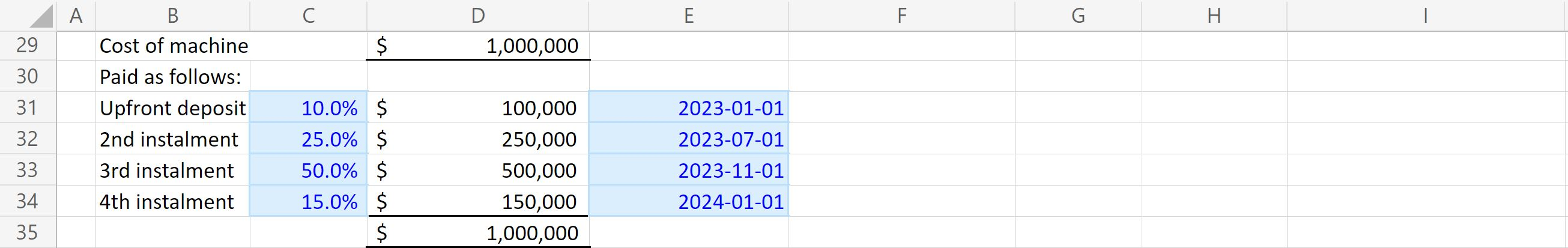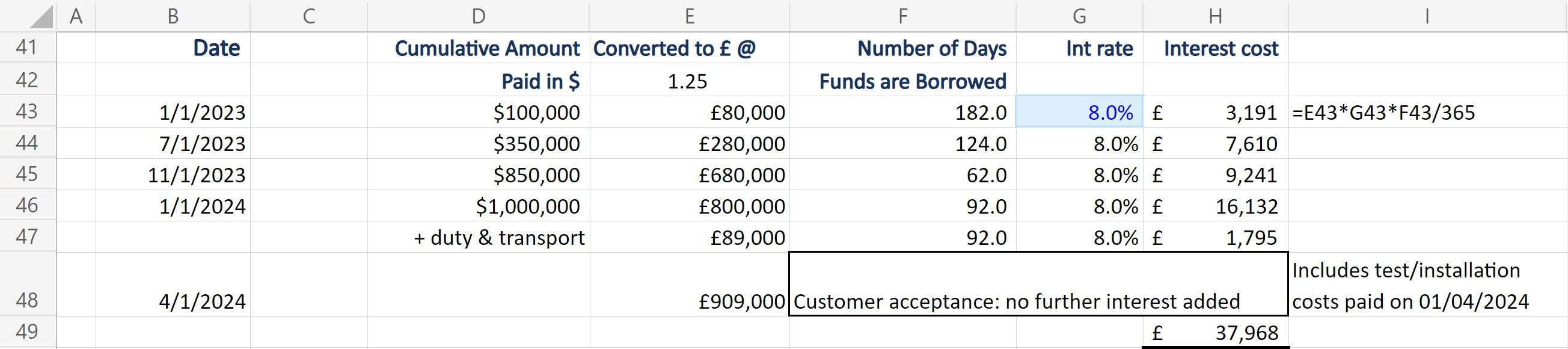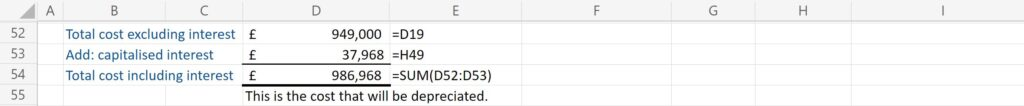## What is Capitalized Interest?

Capitalized interest is interest expense that is incurred while an asset is being developed or constructed and added to the final value of the asset; it will be depreciated over the asset’s expected useful life.

## Key Learning Points

• Capitalized interest is part of an asset’s total cost base.
• The interest to be capitalized must be calculated: add all interest payments made up to the date the asset is ready for use.
• After the asset is entered into use, no more interest is capitalized.

## How Does Capitalized Interest Work?

A fixed asset is one that a business will make use of for more than 12 months. Fixed assets are recorded on the balance sheet and depreciated over the asset’s useful life.

But what cost is recorded as the asset value? For some assets, it is straightforward. For example, you buy a laptop for £600. It would be recorded on the balance sheet at the net cost to the business. If it is a VAT-registered business, it will reclaim £100 VAT (1/6 of 600), so the actual net cost is £500, which is recorded on the balance sheet.

Now consider a more complex asset such as a custom-built machine. In addition to the charge for the machine, there might be delivery and installation costs. If imported, there would be freight and insurance. Depending on its country of origin, customs duties might be charged, and there would likely be testing charges as well.

The machine is custom-built to the buyer’s requirements, so the supplier may require an upfront payment. As the manufacturing process proceeds, instalment payments could be required. Then, the final payment might be made when the machine is shipped from the manufacturer, or when delivered to the customer.

All the interest costs incurred in paying by instalment are also considered to be part of the asset’s cost. If the payments are funded by debt, the interest cost of the financing must be calculated and added to the other components that make up the total asset cost.  Even if no specific debt funding is used to finance the instalments, the cost of using overdraft finance or other revolving credit would be included in the calculation.  The interest cost is added to the other costs, which is called capitalizing the interest.

## When Does the Capitalization Period Start?

When interest costs start to be incurred on payments for the asset, all interest paid until the asset is ready for use is capitalized. Once the asset is ready for use, the capitalization process stops, and no further interest is added to the cost.  This is true even if the asset isn’t used immediately.

To calculate the interest cost:

• calculate the amount and timing of instalments,
• consider the source of funding for the instalments and the cost associated with that funding,
• then calculate the interest cost for the period until the asset is ready for use.

## Example of Capitalized Interest in Excel

Let’s look an example of calculating capitalized interest in Excel, from the cost of the asset, the payment for the asset, and the interest cost.

### Cost of Asset

A machine is purchased for \$1 million. Freight and insurance cost \$50,000. The exchange rate is \$1.25/£1. Customs duties are imposed at 10% on the landed cost. Transport from the port to the end-use site is £5,000. Installation and testing costs are £20,000.

See below how we use this information to calculate the total cost excluding interest below:### Payment for the Asset

Now let’s consider how the machine is paid for. The customer pays a 10% deposit on 1st Jan 2023, another 25% on 1st July 2023, and 50% on completion of manufacture is completed on 1st November 2023. The remaining 15% is paid on delivery at the port of importation, which occurs on 1st January 2024. The customer accepts delivery on 1st April 2024, when testing / installation costs are also paid.### Interest Cost

Now let’s consider the interest cost. The buyer has an overdraft facility, on which is charged 8% interest per annum, and uses this facility to pay the instalments.### Total Cost of the Asset

Calculate the total cost including interest, by adding the total cost excluding interest and the capitalised interest you have calculated.Download the Capitalized Interest Excel files and access the calculation file to see an example of capitalized interest being calculated in Excel in full, from the cost of the asset, to the payment for the asset, through to the interest cost.

## Example of Capitalized Interest

A buyer purchases a custom-built assembly line for £50m. Instalments are due as follows:

1. Upfront deposit 10% on 30th June 2023
2. 2nd instalment of 20% on 30th Sept 2023
3. 3rd instalment of 40% on 31st Dec 2023
4. 4th instalment of 20% on 31st March 2024
5. Balance due on final customer acceptance, 31st Aug 2024.

The installation and testing costs of £5m are all paid on 31st August 2024.

The buyer finances the machine with a new debt facility, drawn down as required to pay the instalments. Interest is charged at 5.75% per annum.

What is the amount of capitalized interest?  What is the total depreciable cost of the asset?

Download the Capitalized Interest Excel files and access the Capitalized Interest Workout Empty file to calculate the interest cost for this scenario, you can then check your working with the Full file.

## Capitalized Interest vs Expensed Interest

Adding interest costs incurred while the asset is being built to the total cost of the asset is ‘Capitalizing Interest’.  If we don’t do that, we would expense the interest – i.e., treat it as a normal business expense in the income statement. That’s what happens after the asset is ready for use. The 2 different treatments are not a choice – capitalizing interest is mandatory while the asset is being built, and expensing the interest is mandatory after the asset becomes useable.

## Capitalized Interest vs Accrued Interest

Accrued interest is the total of interest expenses that have been incurred but not yet formally billed to the borrower. We want to ensure that the financial statements include all interest paid up to the balance sheet date. So, any interest between the date it was last billed, and year-end is calculated and included in the financial statements:

Example – Borrower A has a loan of \$1,000,000 at an interest rate of 8% per annum. Interest is billed by the lender every quarter. A’s year-end is April 30th each year.

Interest will be billed by the lender on 31st March:

\$1,000,000 x 8% x 90 days / 365 = \$19,726.

More interest will be owed, but not yet billed, for the period from 1st April until year-end on 30th April:

\$1,000,000 x 8% x 30 / 365 = \$6,575.

This interest is calculated by A and accrued, or added to A’s liabilities on the balance sheet. This happens regardless of whether the borrowings relate to an asset under construction (the interest would therefore be capitalized) or if the loan is for general corporate purposes (the interest would be expensed in the income statement).

## Conclusion

Capitalized interest is an important part of the total cost of building or developing an asset. Simply total the interest costs from the time of payment until the time the asset is ready for use and add the total interest paid to the total costs of building or buying the asset.

Learn project finance theory, risk management, financing methods, accounting, modeling, return calculation, and apply them practically to build simple and advanced project models with the Project Financier.